# Artin-Rees lemma

View other indispensable lemmata
This article defines a result where the base ring (or one or more of the rings involved) is Noetherian
View more results involving Noetherianness or Read a survey article on applying Noetherianness
This fact is an application of the following pivotal fact/result/idea: Hilbert basis theorem
View other applications of Hilbert basis theorem OR Read a survey article on applying Hilbert basis theorem

## Statement

Suppose$A$ is a Noetherian commutative unital ring and$M$ is a finitely generated$A$-module and$N$ a submodule of$M$.

Suppose: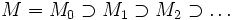$M = M_0 \supset M_1 \supset M_2 \supset \ldots$

is an essentially$I$-adic filtration (in other words, there exists$n_0$ such that for all$n \ge n_0$,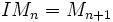$IM_n = M_{n+1}$).

Then the filtration of$N$ given by:$N = N \cap M_0 \supset N \cap M_1 \supset N \cap M_2 \supset \ldots$

is also essentially$I$-adic.

## Proof

### Proof outline

The key idea is the following:

• To any filtration, find an associated module over a Noetherian ring such that the filtration being essentially$I$-adic is equivalent to the module being finitely generated
• Prove that intersecting the filtration with a submodule is equivalent to taking a submodule of the associated module.

### Setting up the modules

Denote by$B_IR$ the blowup algebra of the ideal$I$ in$R$; in other words, the ring: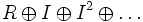$R \oplus I \oplus I^2 \oplus \ldots$

where the multiplication of graded components is just the usual multiplication as elements of <mth>R[/itex].

We now describe a way to associate, to any filtration of a module, an associated module over the blowup algebra. Suppose the filtration is: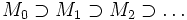$M_0 \supset M_1 \supset M_2 \supset \ldots$

We define the associated module over$B_IR$ as:$B_{\mathcal{F}}(M) = M_0 \oplus M_1 \oplus M_2 \oplus \ldots$

where the multiplication is defined in the usual way.

### The crucial observation

The main step of the proof is to observe that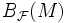$B_{\mathcal{F}}(M)$ is a finitely generated module over$B_IR$ if and only if the filtration of$M$ is essentially$I$-adic.

### Induced filtration on submodule gives submodule on blowup

The module associated for the induced filtration on the submodule$N$ of$M$, is clearly a submodule of the module$B_{\mathcal{F}}(M)$.

### Applying the Hilbert basis theorem

Since$R$ is a Noetherian ring, the ideal$I$ is a finitely generated ideal. Since the blowup algebra is, by construction, generated by its elements of degree zero and one, we see that the blowup algebra is a finitely generated algebra over$R$. Thus, the blowup algebra is a quotient of a polynomial ring over$R$. By the Hilbert basis theorem and the fact that quotients of Noetherian rings are Noetherian, we obtain that$B_IR$ is a Noetherian ring.

Thus, if$B_\mathcal{F}(M)$ is a finitely generated module over$B_IR$, so is the submodule for the induced filtration on$N$.

### Putting the pieces together

Here's the summary of the proof:

• If$\mathcal{F}$ is an essentially$I$-adic filtration on$M$, then the corresponding module$B_{\mathcal{F}}(M)$ is finitely generated over$B_IR$.
• Since$R$ is Noetherian,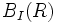$B_I(R)$ is Noetherian.
• The module corresponding to the induced filtration on$N$ is a$B_IR$-submodule of$B_{\mathcal{F}}(M)$. Since the big module is finitely generated and the ring is Noetherian, the submodule is also finitely generated.
• Finally, since the module corresponding to the induced filtration is finitely generated, the induced filtration itself is essentially$I$-adic.